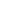# Moving Average Convergence/Divergence - MACD

Moving Average Convergence/Divergence (MACD) is a trend-following momentum indicator that shows the relationship between two moving averages of prices. The MACD is calculated by subtracting the 26-day exponential moving average (EMA) from the 12-day EMA. A nine-day EMA of the MACD, called the "signal line", is then plotted on top of the MACD functioning as a trigger for buy and sell signals. It is better to use this indicator in the situation when the market is fluctuating in a wide range.

The most frequently used MACD signals are the following: crossovers, overbought / oversold phase, and divergence.

The principle of trading with MACD is based on the indicator crossing its signal line. When the MACD falls below the signal line, it is a bearish signal, which indicates that it may be the right time to sell. Conversely, when the MACD rises above the signal line, the indicator gives a bullish signal to buy. Traders also watch for a move above or below the zero line. When the MACD is above zero, it is a signal to buy. The opposite is true when the MACD is below zero which means to sell.

When the MACD rises dramatically, it indicates that a trading instrument is overvalued and overbought and will soon return to realistic values.

When an asset’s price and MACD are not able to reach the same high and a price grows, it means a bullish divergence. In case an asset’s price and MACD fail to hit the same low and a price falls, bearish divergence comes into play.

These divergences are especially important when they arise in overbought / oversold levels.## Calculation

The MACD is calculated by subtracting the value of a 26-period exponential moving average from a 12-period exponential moving average. A 9-period dotted simple moving average of the MACD (the signal line) is then plotted on top of the MACD.

MACD = EMA(CLOSE, 12)-EMA(CLOSE, 26)

SIGNAL = SMA(MACD, 9)

Where:

EMA - the Exponential Moving Average;

SMA - the Simple Moving Average;

SIGNAL - the signal line of the indicator.

All MT5 content